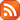Subscribe by RSS

Wed., Dec. 7, 2022 3:30 p.m.

Location: CL 305 and Live Stream

Speaker: Prabhraj Kaur Bains

Title: Modeling of the Neuromuscular Junction Synapse by Probability Distributions (162 kB)Abstract:

The objective is to develop the probability model to compute the probability of the acetylcholine release in neuromuscular synapse under different physiological conditions. The construction of the Inverse Pólya point process model for the probability of the acetylcholine release under a chemical attack. To achieve the goals first, a method of estimation for the number of the acetylcholine vesicles is established. Then, a formula for the probability of the acetylcholine vesicle release in neuromuscular synapse under different conditions ia derived. After that, the axioms for the construction of the Inverse Pólya process model is formulated. Most importantly, the theorem about the probability distribution that satisfy the introduced axioms has been proved. For the case of the chemical effect, a new model, so-called Inverse Pólya process is suggested. A differential equation for happening k events in the Inverse Pólya process is derived.

Live Stream:

https://uregina-ca.zoom.us/j/94125367372